In this article, we will share MP Board Class 10th Maths Book Solutions Chapter 1 Real Numbers Ex 1.2 Pdf, These solutions are solved subject experts from the latest edition books.

## MP Board Class 10th Maths Solutions Chapter 1 Real Numbers Ex 1.2

Question 1.
Express each number as a product of its prime factors:
(i) 140
(ii) 156
(iii) 3825
(iv) 5005
(v) 7429
Solution:
(i) Using factor tree method, we have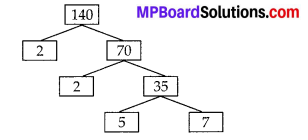∴ 140 = 2 × 2 × 5 × 7 = 22 × 5 × 7

(ii) Using factor tree method, we have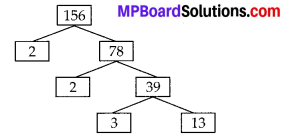∴ 156 = 2 × 2 × 3 × 13 = 22 × 3 × 13

(iii) Using factor tree method, we have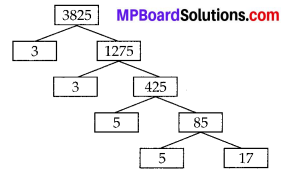3825 = 3 × 3 × 5 × 5 × 17 = 32 × 52 × 17

(iv) Using factor tree method, we have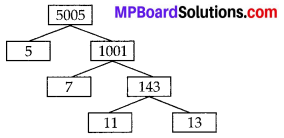∴ 5005 = 5 × 7 × 11 × 13

(v) Using factor tree method, we have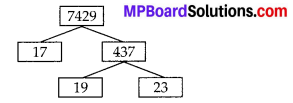∴ 7429 = 17 × 19 × 23

Question 2.
Find the LCM and HCF of the following pairs of integers and verify that LCM × HCF = product of the two numbers.
(i) 26 and 91
(ii) 510 and 92
(iii) 336 and 54
Solution:
(i) Using factor tree method, we have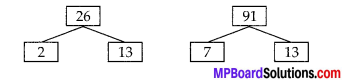⇒ 26 = 2 × 13 and 91 = 7 × 13
LCM of 26 and 91 = 2 × 7 × 13 = 182
HCF of 26 and 91 = 13 Now,
LCM × HCF = 182 × 13 = 2366 and 26 × 91 = 2366
i. e., LCM × HCF = Product of two numbers,

(ii) Using the factor tree method, we have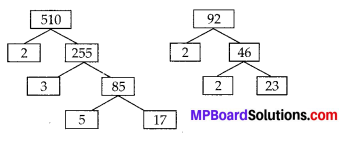⇒ 510 = 2 × 3 × 5 × 17 and 92 = 2 × 2 × 23
∴ LCM of 510 and 92 = 2 × 2 × 3 × 5 × 17 × 23 = 23460
HCF of 510 and 92 = 2 LCM × HCF
= 23460 × 2 = 46920 and 510 × 92 = 46920
i. e., LCM × HCF = Product of two numbers.

(iii) Using the factor tree method, we have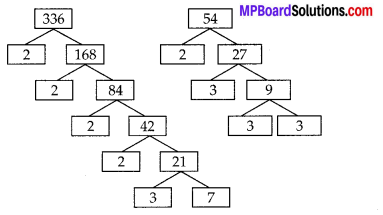336 = 2 × 2 × 2 × 2 × 3 × 7
54 = 2 × 3 × 3 × 3
LCM of 336 and 54
= 2 × 2 × 2 × 2 × 3 × 3 × 3 × 7 = 3024
HCF of 336 and 54 = 2 × 3 = 6
LCM × HCF = 3024 × 6 = 18144
Also 336 × 54 = 18144
Thus, LCM × HCF = Product of two numbers.Question 3.
Find the LCM and HCF of the following integers by applying the prime factorisation method.
(i) 12,15 and 21
(ii) 17,23 and 29
(iii) 8,9 and 25
Solution:
(i) We have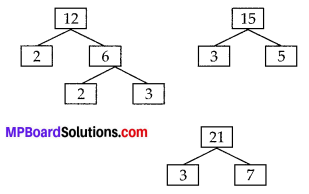⇒ 12 = 2 × 2 × 3, 15 = 3 × 5 and 21 = 3 × 7
∴ HCF of 12, 15 and 21 is 3
LCM of 12,15 and 21 is 2 × 2 × 3 × 5 × 7 i.e., 420

(ii) We have
17 = 1 × 17, 23 = 1 × 23, 29 = 1 × 29
⇒ HCF of 17, 23 and 29 is 1 LCM of 17, 23 and 29 = 17 × 23 × 29 = 11339

(iii) We have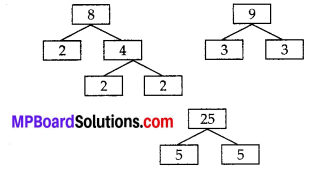⇒ 8 = 2 × 2 × 2, 9 = 3 × 3 and 25 = 5 × 5
∴ HCF of 8, 9 and 25 is 1
LCM of 8, 9 and 25 is 2 × 2 × 2 × 3 × 3 × 5 × 5 = 1800

Question 4.
Given that HCF (306, 657) = 9, find LCM (306, 657).
Solution:
H.C.F × L.C.M = a × b
(a, b) × (a, b) = a × b
9 × x = 306 × 657
x = $$\frac{306 \times 657}{9}$$
∴ x = 22338
∴ L.C.M of (306, 657) = 22338Question 5.
Check whether 6″ can end with the digit 0 for any natural number n.
Solution:
Here, n is a natural number and let 6n ends with digit 0.
∴ 6n is divisible by 5.
But the prime factors of 6 are 2 and 3. i.e.,
6 = 2 × 3
⇒ 6n = (2 × 3)n
i. e., In the prime factorisation of 6n, there is no factor 5.
So, by the fundamental theorem of Arithmetic, every composite number can be expressed as a product of primes and this factorisation is unique apart from the order in which the prime factorisation occurs.
∴ Our assumption that 6n ends with digit 0, is wrong.
Thus, there does not exist any natural number n for which 6n ends with zero.

Question 6.
Explain why 7 × 11 × 13 + 13 and 7 × 6 × 5 × 4 × 3 × 2 × 1 + 5 are composite numbers.
Solution:
i) 7 × 11 × 13 + 13 = 13(77 + 1)
= 13 × 78
∴ It is a composite number
[It is having more than two factors]

ii) 7 × 6 × 5 × 4 × 3 × 2 × 1 + 5
=5[7 × 6 × 4 × 3 × 2 × 1 + 1]
= 5 (1008 + 1)
= 5 × 1009 = 5045
∴ It is a composite number.Question 7.
There is a circular path around a sports field. Sonia takes 18 minutes to drive one round of the field, while Ravi takes 12 minutes for the same. Suppose they both start at the same point and at the same time, and go in the same direction. After how many minutes will they meet again at the starting point?
Solution:
Time taken by Sonia to drive one round of the field = 18 minutes
Time taken by Ravi to drive one round of the field = 12 minutes
The LCM of 18 and 12 gives the exact number of minutes after which they meet again at the starting point.
We have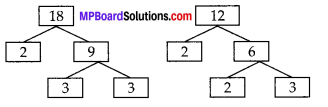⇒ 18 = 2 × 3 × 3 and 12 = 2 × 2 × 3
∴ LCM of 18 and 12 = 2 × 2 × 3 × 3 = 36
Thus, they will meet again at the starting point after 36 minutes.